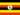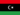La gestion du budgetAll Modules View all topics Close topics
Module 10: Estimation et gestion des coûts### Ce cours a été révisé!

Pour une expérience d'apprentissage plus agréable, nous vous recommandons d'étudier la version mobile relogée de ce cours.

Emmenez-moi au cours révisé.

- or -

Continue studying this course

# La gestion du budget

• Study Reminders

We'll email you at these times to remind you to study

You can set up to 7 reminders per week

#### You're all set

We'll email you at these times to remind you to study

Monday

Tuesday

Wednesday

Thursday

Friday

Saturday

Sunday

• Notes d'étude
• Révisions du sujetOdongo M. 0 0 Managing cash flow if the total money spent on a project is equal to or less than amount budgeted , then the project can still be in troubles if the funding for the project is not available when it is needed and will bring tension between the financial people in an organization. Evaluating The Budget During The Project A project manager must regularly compare the amount of money spent with the budgeted amount and report the information to managers and project stakeholders to establish understanding of how this progress will be measure and reported. Extra money is allocated in a contingency fund to deal with activity where cost exceed estimates. Fund are allocated in a management reserves in case a significant opportunity or challenges occurs that requires change of scope but fund are needed immediately before a scope change can typically be negotiated. The budget cost of work and planned Value The budget cost of work schedule (BCWS) comprises the detailed cost estimates for each activity in the project. The amount of work that should have been done in a particular date is called planned Value (PV). The Plan Value term is used in formula to refer to the sum of the budgeted cost of work up to particular point in the project. Budgeted Cost of Work Performed (BCWP) is the Budgeted Wost of Work Schedule that has been done. If you sum the BCWP value up to certain point in the project schedule , you have the earned value. Earned Value Management (EVM) This method is widely used for medium and high complexity project. (EVM) is the method used for comparing the budget and actual cost of the project periodically during the project and it combine schedule activity with detailed cost estimates for each activities. the Earned value analysis method compare the anticipated cost of work that is schedule to be done at given point in time against what has been done and how much it actually cost. It allow for partial completion of activity if some of the detailed cost associated with activity has been paid and other not. The Actual cost is the amount spent on the items is often more or less that estimated amount in the budget for that items.The Actual cost is the sum of amount actually spent on the items. the Variance Indexes for schedule and the cost is Schedule variance is the different between part of the budget that has been done so far (EV) verses the part that was planned to be completed by now(PV). Similarly the cost variance is the difference between the (EV) and the Actual Cost (AC). Expressed as CV=EV-AC The positive CV value mean that the project is under budget The Schedule Performance Index (SPI) is the ratio of the earned Value and the Planned Value(PV). For example SPI=\$0.62. and EV=\$162.10 and PV=261.65. SPI value less than one mean that the project schedule is behind schedule The formula is SPI= EV/ PV The Cost Performance Index (CPI) is the ratio of the Earned Value (EV) to the Actual Cost (AC) . The formula is CPI = EV/AC. The Formula used to calculate the amount of money needed to complete the project (ETC) depend on whether or not the Cost Variance (CV) to this point is expected to continue (typical)or not (atypical). If the cost Variance is atypical, the (ETC) is simply the original total Budget (BAC) minus the Earned Value (EV) . If they are typical of future.Performance Index (CPI) . Typical cost Variance is caused by the factors that will affect the remaining activity such as high labor and materials cost , then estimate to complete (ETC) needs adjusted by dividing it by the cost performance index (CPI) . For example if labor cost on the first part of a project are estimated at \$80,000 (EV) and Actual Cost (AC) at \$85,000. Typical cost variance to calculate ETC , the project manager must decide if the cost variance observed in the estimate to that point is the representative of the future, for example if the bad weather caused increased in the cost during the part of the project and is likely to have the same effect on the project. if the cost variance up to this point in project is atypical or not typical,then the estimates to complete (ETC) = Budget At Completion (BAC) - Earned Value (EV) ETC= BAC- EV Cost Variance= EV/AV. The cost variance will be \$0.94. To calculate the estimate to complete (ETC)and assuming the cost variance on known activity is typical of future cost, the formula is ETC= ((BAC-EV) / CPI). if the budget at completion (BAC) of the project is \$800,000 and the estimates to complete is (\$ 800,000-\$80000)/\$0.94=\$76,600. Estimate final project cost if the cost of the activity up to the present vary from the original estimates, it will affect the total estimate for the project cost. The new estimate for the project cost is the estimates at the completion (EAC) is the estimates to complete (ETC) added to the Actual Cost (AC) . Formula (EAC) = (ETC) + (AC) .Socorro Z. 0 0 Managing the budget is also important to be able to allocate the scare resources on the basis of forecasted needs for proposed activities over specified period of time. also to coordinate efforts among organizational departments.